Search by Topic

Resources tagged with Symmetry similar to Notes on a Triangle:

Filter by: Content type:
Age range:
Challenge level:

Other tags that relate to Notes on a Triangle
Practical Activity. Reflections. Rotations. Programming. Translations. Animations. Logo. Triangles. Symmetry. STEM - General.

There are 35 results

Broad Topics > Transformations and constructions > SymmetryNotes on a Triangle

Age 11 to 14 Challenge Level:

Can you describe what happens in this film?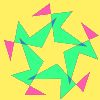Attractive Rotations

Age 11 to 14 Challenge Level:

Here is a chance to create some attractive images by rotating shapes through multiples of 90 degrees, or 30 degrees, or 72 degrees or...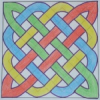Drawing Celtic Knots

Age 11 to 14 Challenge Level:

Here is a chance to create some Celtic knots and explore the mathematics behind them.Flower Power

Age 11 to 16 Challenge Level:

Create a symmetrical fabric design based on a flower motif - and realise it in Logo.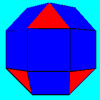Rhombicubocts

Age 11 to 14 Challenge Level:

Each of these solids is made up with 3 squares and a triangle around each vertex. Each has a total of 18 square faces and 8 faces that are equilateral triangles. How many faces, edges and vertices. . . .Two Triangles in a Square

Age 14 to 16 Challenge Level:

Given that ABCD is a square, M is the mid point of AD and CP is perpendicular to MB with P on MB, prove DP = DC.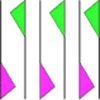Friezes

Age 11 to 14

Some local pupils lost a geometric opportunity recently as they surveyed the cars in the car park. Did you know that car tyres, and the wheels that they on, are a rich source of geometry?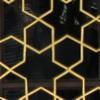Topkapi Palace

Age 7 to 14 Challenge Level:

These images are taken from the Topkapi Palace in Istanbul, Turkey. Can you work out the basic unit that makes up each pattern? Can you continue the pattern? Can you see any similarities and. . . .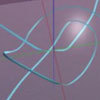Turning Tangles

Age 11 to 14 Challenge Level:

Look carefully at the video of a tangle and explain what's happening.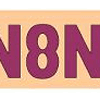Symmetric Trace

Age 14 to 16 Challenge Level:

Points off a rolling wheel make traces. What makes those traces have symmetry?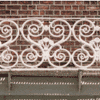Frieze Patterns in Cast Iron

Age 11 to 16

A gallery of beautiful photos of cast ironwork friezes in Australia with a mathematical discussion of the classification of frieze patterns.The Frieze Tree

Age 11 to 16

Patterns that repeat in a line are strangely interesting. How many types are there and how do you tell one type from another?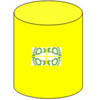Paint Rollers for Frieze Patterns.

Age 11 to 16

Proofs that there are only seven frieze patterns involve complicated group theory. The symmetries of a cylinder provide an easier approach.Age 11 to 14 Challenge Level:

How many different symmetrical shapes can you make by shading triangles or squares?Attractive Tablecloths

Age 14 to 16 Challenge Level:

Charlie likes tablecloths that use as many colours as possible, but insists that his tablecloths have some symmetry. Can you work out how many colours he needs for different tablecloth designs?Gibraltar Geometry

Age 11 to 14 Challenge Level:

Take a look at the photos of tiles at a school in Gibraltar. What questions can you ask about them?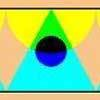One Reflection Implies Another

Age 14 to 16 Challenge Level:

When a strip has vertical symmetry there always seems to be a second place where a mirror line could go. Perhaps you can find a design that has only one mirror line across it. Or, if you thought that. . . .A Roll of Patterned Paper

Age 14 to 16 Challenge Level:

A design is repeated endlessly along a line - rather like a stream of paper coming off a roll. Make a strip that matches itself after rotation, or after reflection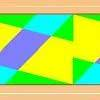Rotations Are Not Single Round Here

Age 14 to 16 Challenge Level:

I noticed this about streamers that have rotation symmetry : if there was one centre of rotation there always seems to be a second centre that also worked. Can you find a design that has only. . . .Overlap

Age 11 to 14 Challenge Level:

A red square and a blue square overlap so that the corner of the red square rests on the centre of the blue square. Show that, whatever the orientation of the red square, it covers a quarter of the. . . .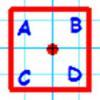Dancing with Maths

Age 7 to 16

An article for students and teachers on symmetry and square dancing. What do the symmetries of the square have to do with a dos-e-dos or a swing? Find out more?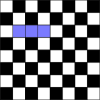Trominoes

Age 11 to 16 Challenge Level:

Can all but one square of an 8 by 8 Chessboard be covered by Trominoes?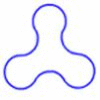Arclets

Age 14 to 16 Challenge Level:

Each of the following shapes is made from arcs of a circle of radius r. What is the perimeter of a shape with 3, 4, 5 and n "nodes".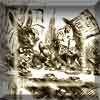A Problem of Time

Age 14 to 16 Challenge Level:

Consider a watch face which has identical hands and identical marks for the hours. It is opposite to a mirror. When is the time as read direct and in the mirror exactly the same between 6 and 7?Reflecting Squarely

Age 11 to 14 Challenge Level:

In how many ways can you fit all three pieces together to make shapes with line symmetry?Sliced

Age 14 to 16 Challenge Level:

An irregular tetrahedron has two opposite sides the same length a and the line joining their midpoints is perpendicular to these two edges and is of length b. What is the volume of the tetrahedron?Pattern Power

Age 5 to 14

Mathematics is the study of patterns. Studying pattern is an opportunity to observe, hypothesise, experiment, discover and create.Prime Magic

Age 7 to 16 Challenge Level:

Place the numbers 1, 2, 3,..., 9 one on each square of a 3 by 3 grid so that all the rows and columns add up to a prime number. How many different solutions can you find?Holly

Age 14 to 16 Challenge Level:

The ten arcs forming the edges of the "holly leaf" are all arcs of circles of radius 1 cm. Find the length of the perimeter of the holly leaf and the area of its surface.Classifying Solids Using Angle Deficiency

Age 11 to 16 Challenge Level:

Toni Beardon has chosen this article introducing a rich area for practical exploration and discovery in 3D geometrySquare Pizza

Age 14 to 16 Challenge Level:

Can you show that you can share a square pizza equally between two people by cutting it four times using vertical, horizontal and diagonal cuts through any point inside the square?Encircling

Age 14 to 16 Challenge Level:

An equilateral triangle is sitting on top of a square. What is the radius of the circle that circumscribes this shape?Troublesome Dice

Age 11 to 14 Challenge Level:

When dice land edge-up, we usually roll again. But what if we didn't...?Tournament Scheduling

Age 11 to 16

Scheduling games is a little more challenging than one might desire. Here are some tournament formats that sport schedulers use.Eight Dominoes

Age 7 to 16 Challenge Level:

Using the 8 dominoes make a square where each of the columns and rows adds up to 8